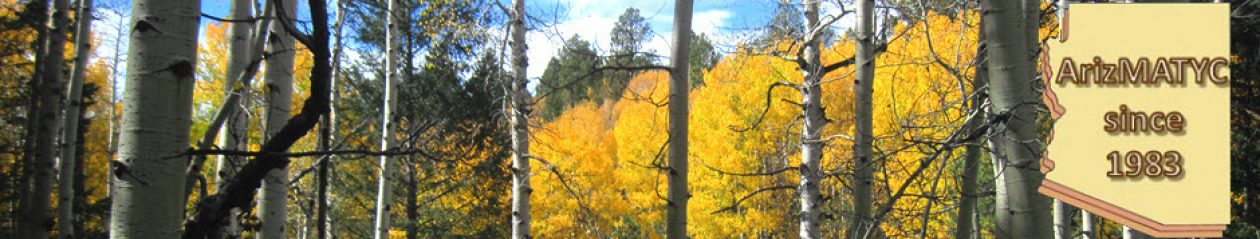ArizMATYC Conference
@ GateWay CC

Friday, October 5, 2018

9:45 a.m. - 10:30 a.m
"Solving Polynomial Equations: Visualization from Linear to Cubic,
from Rational to Complex Numbers "
GeoGebra: https://ggbm.at/ugznkruc

10:45 a.m. to 11:30 a.m
"Making Sense of Integration Visually: Mapping Diagrams for Calculus "

GeoGebra: https://ggbm.at/xkkawf6e

Mapping Diagram Sheets
Mapping Diagram blanks
(2 axis diagrams)
Mapping Diagram blanks
(2 and 3 axes)
MDWorksheet Equations
MDWorksheet Integration
Sections from MD from A B to C and DE (Drafts) Visualizing Functions: An Overview
References
Linear Functions (LF)

GeoGebra (on-line)
Making Sense of Calculus with Mapping Diagrams[2016 MAA]
http://ggbtu.be/bOB534MoK

# Mapping Diagrams to Visualize Complex Analysis

https://ggbm.at/Ni69jyKs